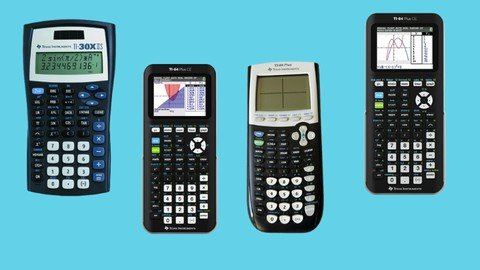﻿ Master The Graphing Calculator Ti-84 Calculator Programming » GFXhome WS

Information of news
27-01-2023, 01:24

### Master The Graphing Calculator Ti-84 Calculator Programming

Category: TutorialsPublished 1/2023
MP4 | Video: h264, 1280x720 | Audio: AAC, 44.1 KHz
Language: English | Size: 703.04 MB | Duration: 0h 42m

Learn Basic Programming Ti-83 or Ti-84 Calculator Skills | Increase your Math Score by Developing Calculator Skills
What you'll learn
Students will learn how to navigate and use the basic functions of the TI-84 calculator, including mathematical operations, data storage, and graphing capabilit
Students will be able to write and execute simple programs in TI-BASIC, including mathematical equations, loops, and branching statements.
Students will learn how to use advanced features of the TI-84, such as matrices, lists, and statistical functions, and how to manipulate and analyze data using
Students will be able to apply their knowledge of the TI-84 to real-world problem-solving, such as solving equations and modeling data in various fields like ma
Requirements
No programming experience is needed. We will teach you how to code.
Description
In this course, students will learn how to effectively use and program the TI-84 calculator. The course will cover a wide range of topics, including basic calculator functions, programming in TI-BASIC, and advanced features such as matrices and statistical functions.Throughout the course, students will be introduced to various concepts and techniques that will help them to understand the capabilities of the TI-84 calculator. They will learn how to navigate and use the basic functions of the calculator, including mathematical operations, data storage, and graphing capabilities.In addition, students will gain hands-on experience in writing and executing simple programs in TI-BASIC, including mathematical equations, loops, and branching statements. This will enable them to create their own programs and solve problems on the calculator.The course will also cover advanced features of the TI-84, such as matrices, lists, and statistical functions, and how to manipulate and analyze data using these tools. This will give students a more in-depth understanding of the calculator's capabilities and will allow them to apply their knowledge to real-world problem-solving in various fields like math, science, and engineering.By the end of the course, students will be able to use the TI-84 calculator with confidence and proficiency and will be able to apply their knowledge to solve problems in their studies and future careers.There are several different versions of the Texas Instruments Graphing Calculator that I use in this course, including the following:TI-83 PlusTI-84 PlusTI-84 Plus Silver EditionTI-84 Plus C Silver EditionTI-84 Plus CE
Overview
Section 1: Getting Started
Lecture 1 Introduction
Section 2: Learn how to program!
Lecture 2 Activating Lowercase
Lecture 3 Distance Formula
Lecture 5 Primality Test
Lecture 6 Binomial Expansion
Lecture 8 Polynomial Multiplication
Lecture 9 Systems of three equations
Beginners wanting to learn how to code use their TI-83 or TI-84 calculators
ScreenshotsSite BBcode/HTML Code:
Dear visitor, you went to the site as unregistered user.